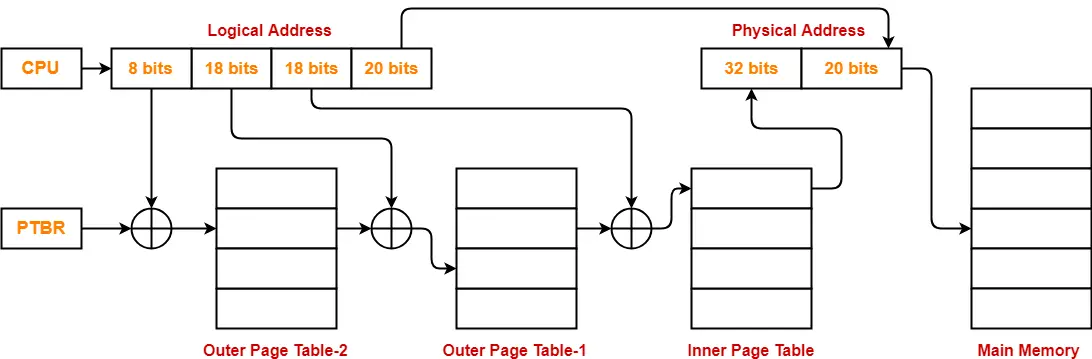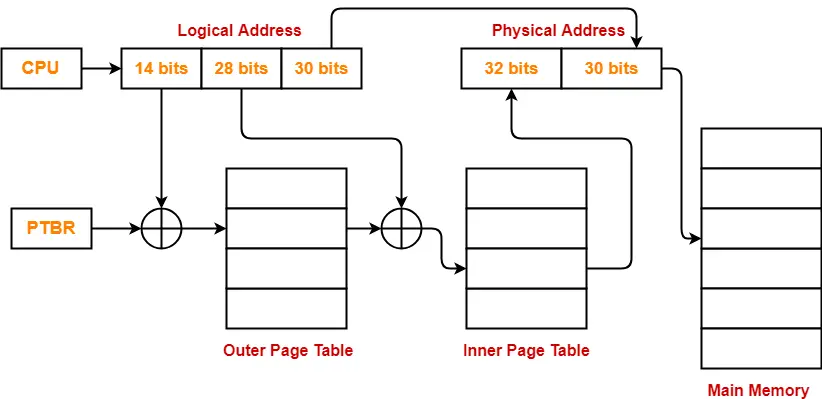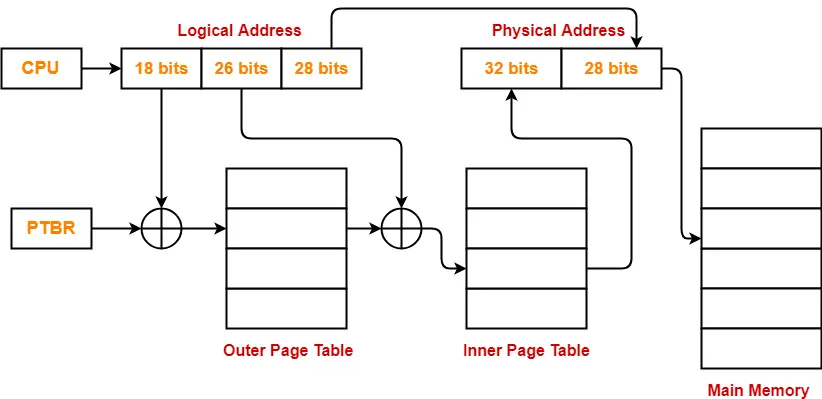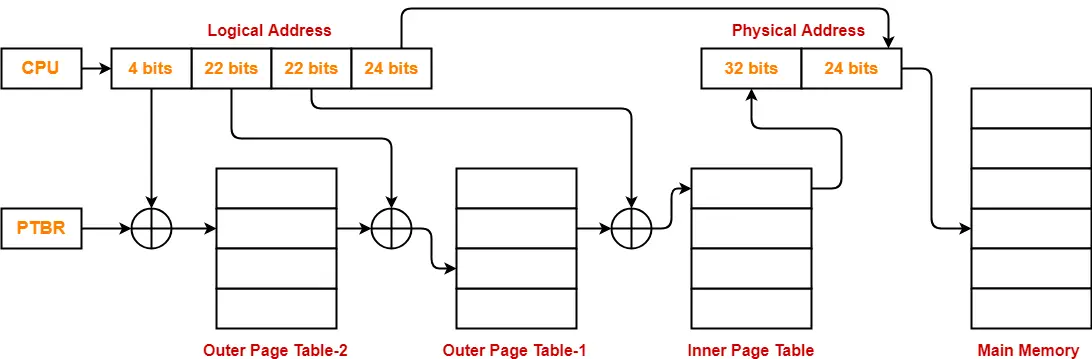# Multilevel Paging | Paging | Practice Problems

## Multilevel Paging in OS-

Before you go through this article, make sure that you have gone through the previous article on Multilevel Paging.

We have discussed-

• Multilevel Paging is a paging scheme where there exists a hierarchy of Page Tables.
• Multilevel paging is needed when a page table can not be stored in a single frame due to its large size.

In this article, we will discuss practice problems based on multilevel paging.

## Problem-01:

Consider a system using multilevel paging scheme. The page size is 1 MB. The memory is byte addressable and virtual address is 64 bits long. The page table entry size is 4 bytes.

Find-

1. How many levels of page table will be required?

## Solution-

Given-

• Virtual Address = 64 bits
• Page size = 1 MB
• Page table entry size = 4 bytes

### Number of Bits in Frame Number-

We have,

Page table entry size

= 4 bytes

= 32 bits

Thus, Number of bits in frame number = 32 bits

### Number of Frames in Main Memory-

We have, Number of bits in frame number = 32 bits

Thus,

Number of frames in main memory

= 232 frames

### Size of Main Memory-

Size of main memory

= Total number of frames x Frame size

= 232 x 1 MB

= 252 B

Thus, Number of bits in physical address = 52 bits

### Number of Bits in Page Offset-

We have,

Page size

= 1 MB

= 220 B

Thus, Number of bits in page offset = 20 bits

Alternatively,

Number of bits in page offset

= Number of bits in physical address – Number of bits in frame number

= 52 bits – 32 bits

= 20 bits

### Process Size-

Number of bits in virtual address = 64 bits

Thus,

Process size

= 264 bytes

### Number of Pages of Process-

Number of pages the process is divided

= Process size / Page size

= 264 B / 1 MB

= 264 B / 220 B

= 244 pages

### Inner Page Table Size-

Inner page table keeps track of the frames storing the pages of process.

Inner page table size

= Number of entries in inner page table x Page table entry size

= Number of pages the process is divided x Page table entry size

= 244 x 4 bytes

= 246 bytes

Now, we can observe-

• The size of inner page table is greater than the frame size (1 MB).
• Thus, inner page table can not be stored in a single frame.
• So, inner page table has to be divided into pages.

### Number of Pages of Inner Page Table-

Number of pages the inner page table is divided

= Inner page table size / Page size

= 246 B / 1 MB

= 246 B / 220 B

= 226 pages

Now, these 226 pages of inner page table are stored in different frames of the main memory.

### Number of Page Table Entries in One Page of Inner Page Table-

Number of page table entries in one page of inner page table

= Page size / Page table entry size

= 1 MB / 4 B

= 220 B / 22 B

= 218 entries

### Number of Bits Required to Search an Entry in One Page of Inner Page Table-

One page of inner page table contains 218 entries.

Thus,

Number of bits required to search a particular entry in one page of inner page table = 18 bits

### Outer Page Table-1 Size-

Outer page table-1 is required to keep track of the frames storing the pages of inner page table.

Outer page table-1 size

= Number of entries in outer page table-1 x Page table entry size

= Number of pages the inner page table is divided x Page table entry size

= 226 x 4 bytes

= 228 bytes

= 256 MB

Now, we can observe-

• The size of outer page table-1 is greater than the frame size (1 MB).
• Thus, outer page table-1 can not be stored in a single frame.
• So, outer page table-1 has to be divided into pages.

### Number of Pages of Outer Page Table-1

Number of pages the outer page table-1 is divided

= Outer page table-1 size / Page size

= 256 MB / 1 MB

= 256 pages

Now, these 256 pages of outer page table-1 are stored in different frames of the main memory.

### Number of Page Table Entries in One Page of Outer Page Table-1

Number of page table entries in one page of outer page table-1

= Page size / Page table entry size

= 1 MB / 4 B

= 220 B / 22 B

= 218 entries

### Number of Bits Required to Search an Entry in One Page of Outer Page Table-1

One page of outer page table-1 contains 218 entries.

Thus,

Number of bits required to search a particular entry in one page of outer page table-1 = 18 bits

### Outer Page Table-2 Size-

Outer page table-2 is required to keep track of the frames storing the pages of outer page table-1.

Outer page table-2 size

= Number of entries in outer page table-2 x Page table entry size

= Number of pages the outer page table-1 is divided x Page table entry size

= 256 x 4 bytes

= 1 KB

Now, we can observe-

• The size of outer page table-2 is less than the frame size (16 KB).
• Thus, outer page table-2 can be stored in a single frame.
• In fact, outer page table-2 will not completely occupy one frame and some space will remain vacant.
• So, for given system, we will have three levels of page table.
• Page Table Base Register (PTBR) will store the base address of the outer page table-2.

### Number of Bits Required to Search an Entry in Outer Page Table-2

Outer page table-2 contains 256 = 28 entries.

Thus,

Number of bits required to search a particular entry in outer page table-2 = 8 bits

The paging system will look like as shown below-## Problem-02:

Consider a system using multilevel paging scheme. The page size is 1 GB. The memory is byte addressable and virtual address is 72 bits long. The page table entry size is 4 bytes.

Find-

1. How many levels of page table will be required?

## Solution-

Given-

• Virtual Address = 72 bits
• Page size = 1 GB
• Page table entry size = 4 bytes

### Number of Bits in Frame Number-

We have,

Page table entry size

= 4 bytes

= 32 bits

Thus, Number of bits in frame number = 32 bits

### Number of Frames in Main Memory-

We have, Number of bits in frame number = 32 bits

Thus,

Number of frames in main memory

= 232 frames

### Size of Main Memory-

Size of main memory

= Total number of frames x Frame size

= 232 x 1 GB

= 262 B

Thus, Number of bits in physical address = 62 bits

### Number of Bits in Page Offset-

We have,

Page size

= 1 GB

= 230 B

Thus, Number of bits in page offset = 30 bits

Alternatively,

Number of bits in page offset

= Number of bits in physical address – Number of bits in frame number

= 62 bits – 32 bits

= 30 bits

### Process Size-

Number of bits in virtual address = 72 bits

Thus,

Process size

= 272 bytes

### Number of Pages of Process-

Number of pages the process is divided

= Process size / Page size

= 272 B / 1 GB

= 272 B / 230 B

= 242 pages

### Inner Page Table Size-

Inner page table keeps track of the frames storing the pages of process.

Inner page table size

= Number of entries in inner page table x Page table entry size

= Number of pages the process is divided x Page table entry size

= 242 x 4 bytes

= 244 bytes

Now, we can observe-

• The size of inner page table is greater than the frame size (1 GB).
• Thus, inner page table can not be stored in a single frame.
• So, inner page table has to be divided into pages.

### Number of Pages of Inner Page Table-

Number of pages the inner page table is divided

= Inner page table size / Page size

= 244 B / 1 GB

= 244 B / 230 B

= 214 pages

Now, these 214 pages of inner page table are stored in different frames of the main memory.

### Number of Page Table Entries in One Page of Inner Page Table-

Number of page table entries in one page of inner page table

= Page size / Page table entry size

= 1 GB / 4 B

= 230 B / 22 B

= 228 entries

### Number of Bits Required to Search an Entry in One Page of Inner Page Table-

One page of inner page table contains 228 entries.

Thus,

Number of bits required to search a particular entry in one page of inner page table = 28 bits

### Outer Page Table Size-

Outer page table is required to keep track of the frames storing the pages of inner page table.

Outer page table size

= Number of entries in outer page table x Page table entry size

= Number of pages the inner page table is divided x Page table entry size

= 214 x 4 bytes

= 216 bytes

= 64 KB

Now, we can observe-

• The size of outer page table is less than the frame size (1 GB).
• Thus, outer page table can be stored in a single frame.
• In fact, outer page table will not completely occupy one frame and some space will remain vacant.
• So, for given system, we will have two levels of page table.
• Page Table Base Register (PTBR) will store the base address of the outer page table.

### Number of Bits Required to Search an Entry in Outer Page Table-

Outer page table contains 214 entries.

Thus,

Number of bits required to search a particular entry in outer page table = 14 bits

The paging system will look like as shown below-## Problem-03:

Consider a system using multilevel paging scheme. The page size is 256 MB. The memory is byte addressable and virtual address is 72 bits long. The page table entry size is 4 bytes.

Find-

1. How many levels of page table will be required?

## Solution-

Given-

• Virtual Address = 72 bits
• Page size = 256 MB
• Page table entry size = 4 bytes

### Number of Bits in Frame Number-

We have,

Page table entry size

= 4 bytes

= 32 bits

Thus, Number of bits in frame number = 32 bits

### Number of Frames in Main Memory-

We have, Number of bits in frame number = 32 bits

Thus,

Number of frames in main memory

= 232 frames

### Size of Main Memory-

Size of main memory

= Total number of frames x Frame size

= 232 x 256 MB

= 260 B

Thus, Number of bits in physical address = 60 bits

### Number of Bits in Page Offset-

We have,

Page size

= 256 MB

= 228 B

Thus, Number of bits in page offset = 28 bits

Alternatively,

Number of bits in page offset

= Number of bits in physical address – Number of bits in frame number

= 60 bits – 32 bits

= 28 bits

### Process Size-

Number of bits in virtual address = 72 bits

Thus,

Process size

= 272 bytes

### Number of Pages of Process-

Number of pages the process is divided

= Process size / Page size

= 272 B / 256 MB

= 272 B / 228 B

= 244 pages

### Inner Page Table Size-

Inner page table keeps track of the frames storing the pages of process.

Inner page table size

= Number of entries in inner page table x Page table entry size

= Number of pages the process is divided x Page table entry size

= 244 x 4 bytes

= 246 bytes

Now, we can observe-

• The size of inner page table is greater than the frame size (256 MB).
• Thus, inner page table can not be stored in a single frame.
• So, inner page table has to be divided into pages.

### Number of Pages of Inner Page Table-

Number of pages the inner page table is divided

= Inner page table size / Page size

= 246 B / 256 MB

= 246 B / 228 B

= 218 pages

Now, these 218 pages of inner page table are stored in different frames of the main memory.

### Number of Page Table Entries in One Page of Inner Page Table-

Number of page table entries in one page of inner page table

= Page size / Page table entry size

= 256 MB / 4 B

= 228 B / 22 B

= 226 entries

### Number of Bits Required to Search an Entry in One Page of Inner Page Table-

One page of inner page table contains 226 entries.

Thus,

Number of bits required to search a particular entry in one page of inner page table = 26 bits

### Outer Page Table Size-

Outer page table is required to keep track of the frames storing the pages of inner page table.

Outer page table size

= Number of entries in outer page table x Page table entry size

= Number of pages the inner page table is divided x Page table entry size

= 218 x 4 bytes

= 220 bytes

= 1 MB

Now, we can observe-

• The size of outer page table is less than the frame size (256 MB).
• Thus, outer page table can be stored in a single frame.
• In fact, outer page table will not completely occupy one frame and some space will remain vacant.
• So, for given system, we will have two levels of page table.
• Page Table Base Register (PTBR) will store the base address of the outer page table.

### Number of Bits Required to Search an Entry in Outer Page Table-

Outer page table contains 218 entries.

Thus,

Number of bits required to search a particular entry in outer page table = 18 bits

The paging system will look like as shown below-## Problem-04:

Consider a system using multilevel paging scheme. The page size is 16 MB. The memory is byte addressable and virtual address is 72 bits long. The page table entry size is 4 bytes.

Find-

1. How many levels of page table will be required?

## Solution-

Given-

• Virtual Address = 72 bits
• Page size = 16 MB
• Page table entry size = 4 bytes

### Number of Bits in Frame Number-

We have,

Page table entry size

= 4 bytes

= 32 bits

Thus, Number of bits in frame number = 32 bits

### Number of Frames in Main Memory-

We have, Number of bits in frame number = 32 bits

Thus,

Number of frames in main memory

= 232 frames

### Size of Main Memory-

Size of main memory

= Total number of frames x Frame size

= 232 x 16 MB

= 256 B

Thus, Number of bits in physical address = 56 bits

### Number of Bits in Page Offset-

We have,

Page size

= 16 MB

= 224 B

Thus, Number of bits in page offset = 24 bits

Alternatively,

Number of bits in page offset

= Number of bits in physical address – Number of bits in frame number

= 56 bits – 32 bits

= 24 bits

### Process Size-

Number of bits in virtual address = 72 bits

Thus,

Process size

= 272 bytes

### Number of Pages of Process-

Number of pages the process is divided

= Process size / Page size

= 272 B / 16 MB

= 272 B / 224 B

= 248 pages

### Inner Page Table Size-

Inner page table keeps track of the frames storing the pages of process.

Inner page table size

= Number of entries in inner page table x Page table entry size

= Number of pages the process is divided x Page table entry size

= 248 x 4 bytes

= 250 bytes

Now, we can observe-

• The size of inner page table is greater than the frame size (16 MB).
• Thus, inner page table can not be stored in a single frame.
• So, inner page table has to be divided into pages.

### Number of Pages of Inner Page Table-

Number of pages the inner page table is divided

= Inner page table size / Page size

= 250 B / 16 MB

= 250 B / 224 B

= 226 pages

Now, these 226 pages of inner page table are stored in different frames of the main memory.

### Number of Page Table Entries in One Page of Inner Page Table-

Number of page table entries in one page of inner page table

= Page size / Page table entry size

= 16 MB / 4 B

= 224 B / 22 B

= 222 entries

### Number of Bits Required to Search an Entry in One Page of Inner Page Table-

One page of inner page table contains 222 entries.

Thus,

Number of bits required to search a particular entry in one page of inner page table = 22 bits

### Outer Page Table-1 Size-

Outer page table-1 is required to keep track of the frames storing the pages of inner page table.

Outer page table-1 size

= Number of entries in outer page table-1 x Page table entry size

= Number of pages the inner page table is divided x Page table entry size

= 226 x 4 bytes

= 228 bytes

= 256 MB

Now, we can observe-

• The size of outer page table-1 is greater than the frame size (16 MB).
• Thus, outer page table-1 can not be stored in a single frame.
• So, outer page table-1 has to be divided into pages.

### Number of Pages of Outer Page Table-1

Number of pages the outer page table-1 is divided

= Outer page table-1 size / Page size

= 256 MB / 16 MB

= 16 pages

Now, these 16 pages of outer page table-1 are stored in different frames of the main memory.

### Number of Page Table Entries in One Page of Outer Page Table-1

Number of page table entries in one page of outer page table-1

= Page size / Page table entry size

= 16 MB / 4 B

= 224 B / 22 B

= 222 entries

### Number of Bits Required to Search an Entry in One Page of Outer Page Table-1

One page of outer page table-1 contains 222 entries.

Thus,

Number of bits required to search a particular entry in one page of outer page table-1 = 22 bits

### Outer Page Table-2 Size-

Outer page table-2 is required to keep track of the frames storing the pages of outer page table-1.

Outer page table-2 size

= Number of entries in outer page table-2 x Page table entry size

= Number of pages the outer page table-1 is divided x Page table entry size

= 16 x 4 bytes

= 64 bytes

Now, we can observe-

• The size of outer page table-2 is less than the frame size (16 MB).
• Thus, outer page table-2 can be stored in a single frame.
• In fact, outer page table-2 will not completely occupy one frame and some space will remain vacant.
• So, for given system, we will have three levels of page table.
• Page Table Base Register (PTBR) will store the base address of the outer page table-2.

### Number of Bits Required to Search an Entry in Outer Page Table-2

Outer page table-2 contains 16 = 24 entries.

Thus,

Number of bits required to search a particular entry in outer page table-2 = 4 bits

The paging system will look like as shown below-Next Article- Practice Problems On Multilevel Paging | Set-02

Get more notes and other study material of Operating System.

Watch video lectures by visiting our YouTube channel LearnVidFun.

SummaryArticle Name
Multilevel Paging | Paging | Practice Problems
Description
Practice Problems based on Multilevel Paging in OS. Multilevel paging is a paging scheme where there exists a hierarchy of page tables. Multilevel Paging is needed when the size of page table is greater than the frame size.
Author
Publisher Name
Gate Vidyalay
Publisher Logo